Include Top

# Case Study 3 – Six Sigma DMAIC Project Portfolio Selection

1. Open the workbook DMAIC Project Selection - Optimization. DiscoverSim Input Distributions will simulate the variability in the cost savings and probability of success for each project. We will specify cost savings as a Triangular Distribution for each project in cells G14 to G22. The probability of success will be specified at cells K14 to K22 using the Bernoulli (Yes/No) Distribution.

2.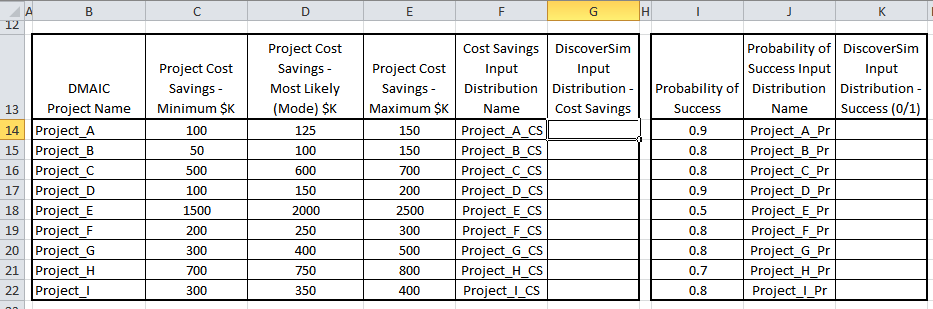3. DiscoverSim Input Controls (Discrete) will be used to “select” the project (0,1) and specified in cells O14 to O22.

4.5. Click on cell G14 to specify the “Cost Savings” Input Distribution for Project_A. Select DiscoverSim > Input Distribution:

6.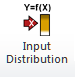7. Select Triangular Distribution.

8. Click the input name cell reference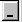and specify cell F14 containing the input Name “Project_A_CS” (denoting “Project A Cost Savings”). After specifying a cell reference, the dropdown symbol changes fromto.

9. Click the Minimum parameter cell referenceand specify cell C14 containing the minimum parameter value = 100 (\$K).

10. Click the Mode parameter cell referenceand specify cell D14 containing the mode (most likely) parameter value = 125 (\$K).

11. Click the Maximum parameter cell referenceand specify cell E14 containing the maximum parameter value = 150 (\$K).

12. Click Update Chart to view the Triangular Distribution as shown:

13.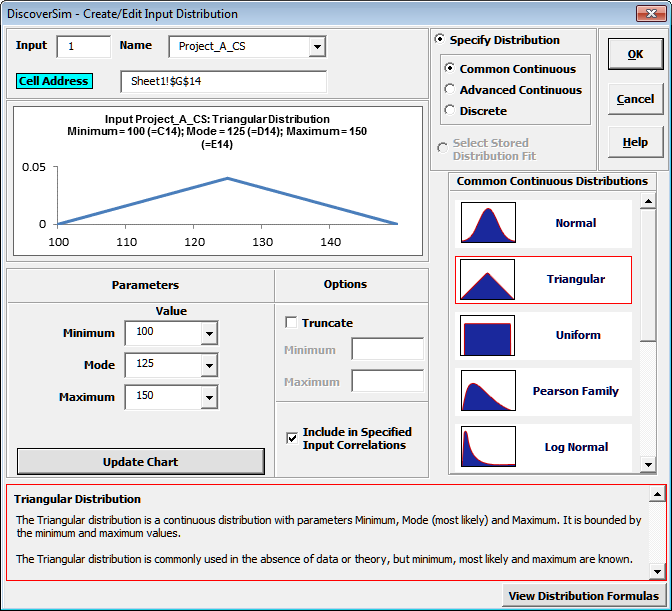14. Click OK. Hover the cursor on cell G14 to view the DiscoverSim graphical comment showing the distribution and parameter values:

15.16. Click on cell G14. Click the DiscoverSim Copy Cell menu button(Do not use Excel’s Copy – it will not work!).

17. Select cells G15:G22. Now click the DiscoverSim Paste Cell menu button( Do not use Excel’s Paste – it will not work!).

18. Review the input comments in cells G15 to G22.

19. Click on cell K14 to specify the “Probability of Success” Input Distribution for Project_A. Select DiscoverSim > Input Distribution:

20.21. Select Discrete and Bernoulli Distribution. Bernoulli is also sometimes referred to as the “Yes/No” distribution.

22. Click the input Name cell reference and specify cell J14 containing the input name “Project_A_Pr” (denoting “Project A Probability of Success”).

23. Click the Event Probability parameter cell reference and specify cell I14 containing the probability parameter value = 0.9.

24. Click Update Chart to view the Bernoulli distribution as shown:

25.26. Click OK. Hover the cursor on cell K14 to view the DiscoverSim graphical comment showing the distribution and parameter values:

27.28. Click on cell K14. Click the DiscoverSim Copy Cell menu button(Do not use Excel’s Copy – it will not work!).

29. Select cells K15:K22. Now click the DiscoverSim Paste Cell menu button(Do not use Excel’s Paste – it will not work!).

30. Review the input comments in cells K15 to K22.

31. Now we will add Discrete Input Controls (also referred to as “Decision Variables”). These will be used to “select” the project (0 denotes project not selected, 1 denotes project selected). DiscoverSim will use stochastic global optimization to determine which projects to select and which not to select in order to maximize total cost savings. A constraint will also be added to ensure that the total number of resources is <=2 0.

32. Click on cell O14. Select Control:33. Click input Name cell referenceand specify cell N14 containing the input control name “Project_A_Select”.

34. Select Discrete (this input control setting will consider only integer values). Set the Max value to 1 as shown:

35.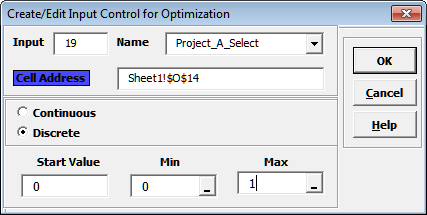36. Click OK. Hover the cursor on cell O14 to view the comment displaying the Input Control settings:

37.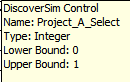38. Click on cell O14. Click the DiscoverSim Copy Cell menu button(Do not use Excel’s Copy – it will not work!).

39. Select cells O15:O22. Click the DiscoverSim Paste Cell menu button(Do not use Excel’s Paste – it will not work!).

40. Review the input control comments in cells O15 to O22.

41. Now we will add the constraint. Click on cell O26. Select Constraint: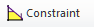42. Enter the Constraint Name as “Resource_Constraint”. Enter O24 in the “Left Hand Side” (LHS) or click the LHS cell referenceand select O24. Select <=. Enter 20 in the “Right Hand Side” (RHS).
43.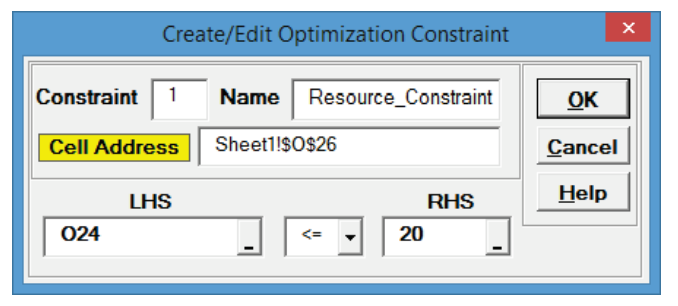Note: Cell O24 contains the Excel formula: =SUMPRODUCT(O14:O22,M14:M22). This sums the product of resources*selected(0,1), in other words the sum of selected project resources.

44. Click OK. Review the comment at cell O26.

45.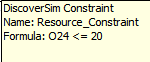The cell display is “TRUE” since the initial value for O24 is zero which obviously satisfies the constraint.

46. Now we will specify the output. Click on cell Q24. The cell contains the Excel formula for the sum of expected savings as the sum of: Cost Savings * Probability of Success (if project is selected) or 0 (if project is not selected).

47. Select DiscoverSim > Output Response:

48.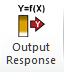49. Enter the output Name as “Total_Savings”. Enter the Lower Specification Limit (LSL) as 1000. The Weight and Output Goal settings are used only for multiple response optimization, so do not need to be modified in this example.
50.Click OK.

51. Hover the cursor on cell Q24 to view the DiscoverSim Output information.

52.53. Now we are ready to perform the optimization to select projects. Select DiscoverSim > Run Optimization:

54.55. Select “Maximize” for Optimization Goal and “Mean’ for Statistic. Select Seed Value and enter “12” in order to replicate the optimization results given below. Change Replications to 1000 to reduce the optimization time. All other settings will be default as shown:

56.Note: Maximize Mean may not produce the lowest possible standard deviation, so we will consider Maximize Ppl later.

We will use the Hybrid optimization method which requires more time to compute, but is very powerful to solve complex optimization problems. Since this is a small discrete problem, Discrete Exhaustive will be used. If there were more projects, say 20, then MIDACO would have been used

57. Click Run.

58. The final optimal parameter values are given as:

59.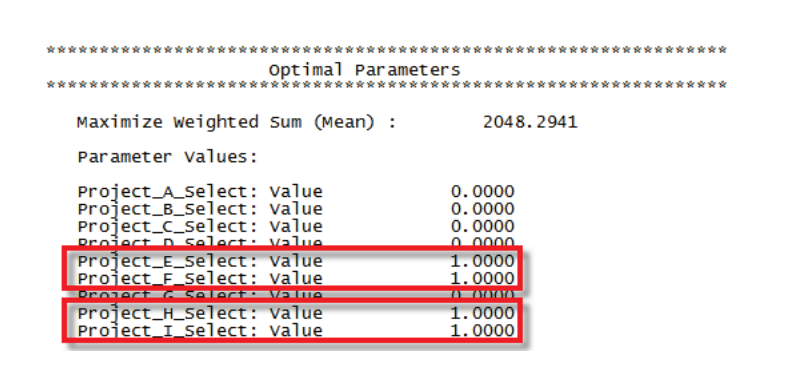Projects E, F, H and I have been selected giving a predicted mean cost savings of 2004 (\$K). Intuitively, these would be the projects one would select given that they have the highest expected values per resource. However, a simple “pick the winner” approach fails to take into account the variability and likelihood of meeting the minimum total cost saving requirement of 1000 \$K.

60. The constraint equation evaluated at this optimum has been satisfied (amount violated = 0):

61.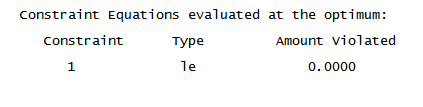Project E requires 10 resources, F requires 2, H requires 5 and I requires 3 for a total of 20, satisfying our constraint that the total resources be less than or equal to 20.

62. You are prompted to paste the optimal values into the spreadsheet:
63.Click Yes. This sets the Input Control values to the optimum values as shown:64. Select DiscoverSim > Run Simulation:

65.66. Select Seed Value and enter “123” as shown, in order to replicate the simulation results given below.

67.Click Run.

68. The DiscoverSim Output Report displays a histogram, descriptive statistics and process capability indices:

69.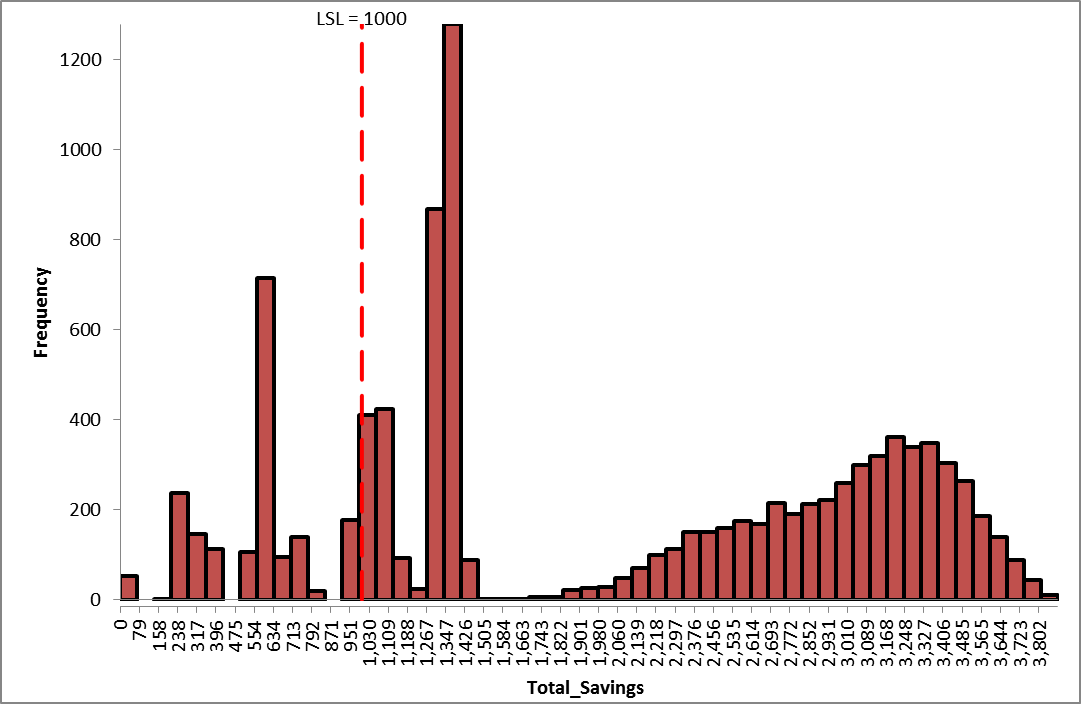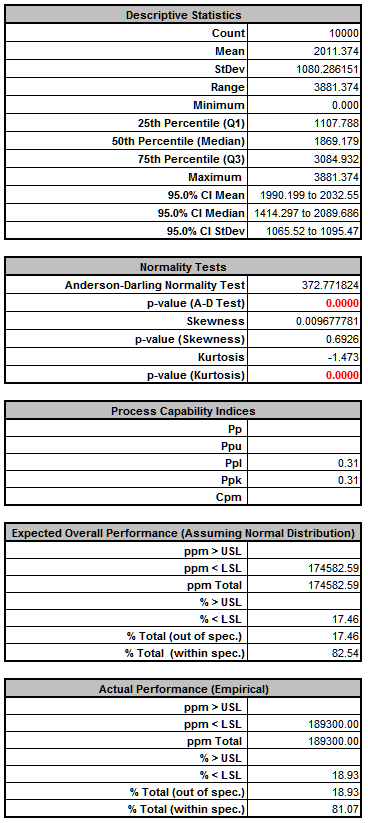From the histogram and capability report we see that the expected mean total savings are good (2011 \$K), but the standard deviation is very large (1080 \$K). Looking at the Actual Performance report we see that there is an 18.9% chance of failing to meet the management requirement of 1000 \$K.

70. Now we will rerun the optimization to select projects, but this time, we will maximize the process capability index Ppl. (Ppl is used since we only have a lower specification limit). This will simultaneously maximize the mean and minimize the standard deviation:

71. Ppl = (Mean – LSL)/(3*StDev).

An equivalent alternative would be to minimize Calculated DPM.

Note: A nonparametric alternative statistic would be %Ppl (percentile Ppl):

%Ppl = (Median – LSL)/(Median - 0.135 Percentile).

This is robust to the assumption of normality. Another robust statistic would be Actual DPM. With either of these we would recommend increasing the number of replications to 10,000 to improve the precision of the statistic, but this would require significantly more computation time for optimization.

72. Reset the project selection by changing the Input Control values to 0:

73.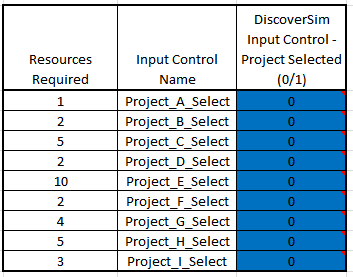74. Select DiscoverSim > Run Optimization

75.76. Select “Maximize” for Optimization Goal, “Weighted Sum” for Multiple Output Metric and “PpL” for Statistic. Select Seed Value and enter “12”, in order to replicate the optimization results given below. Set Replications to 1000 to reduce the optimization time. All other settings will be default as shown:
77.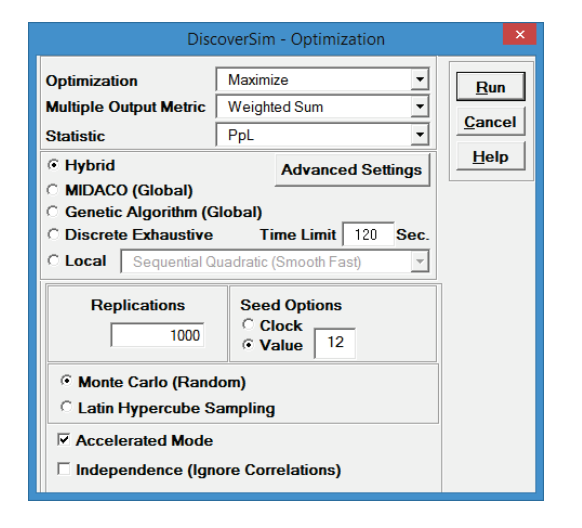Click Run.

78. The final optimal parameter values are given as:

79.Projects A, C, F, G, H, and I have been selected giving a predicted Ppl of 0.62.

Note: Project E, previously selected to maximize the mean, was not selected when the goal was to maximize the process capability, Ppl.

The constraint requirement that the total number of resources be <=2 0 is satisfied with this selection.

80. You are prompted to paste the optimal values into the spreadsheet:
81.Click Yes. This sets the Input Control values to the optimum values as shown: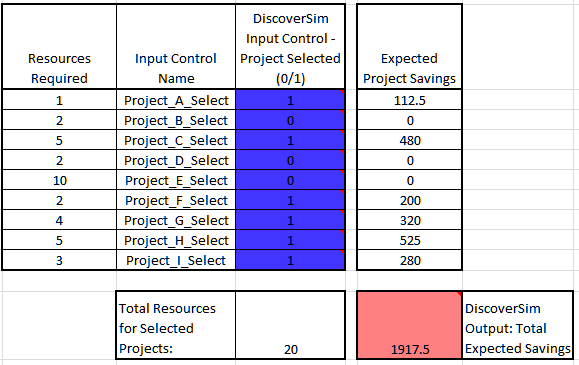82. Select DiscoverSim > Run Simulation:

83.84. Select Seed Value and enter “12” as shown, in order to replicate the simulation results given below.

85.Click Run.

86. The DiscoverSim Output Report displays a histogram, descriptive statistics and process capability indices:

87.Note that the mean total savings have been reduced somewhat from 2011 to 1925 \$K, however, the standard deviation has been reduced from 1080 to 484 \$K and the chance of failing to meet the management requirement of 1000 \$K has been reduced from 18.9% to 4.0% (actual performance).

Considering the two selection scenarios, one can present the risks to management and make the project selection decision to go either way: take the risk and maximize the mean with potential larger savings, or choose the safer projects that result in less variation and significantly improve the chance of meeting a minimum savings of 1 million.

88. Clearly, Project E is the key project to consider in this analysis. The impact of this project can be confirmed with a sensitivity analysis.

89. Set all of the Input Control values to 1 as shown:

90.91. Select DiscoverSim > Run Simulation:

92.93. Click Report Options/Sensitivity Analysis. Check Sensitivity Regression Analysis, Sensitivity Charts - Correlation Coefficients and Regression Coefficients. Select Seed Value and enter “12” as shown, in order to replicate the simulation results given below.

94.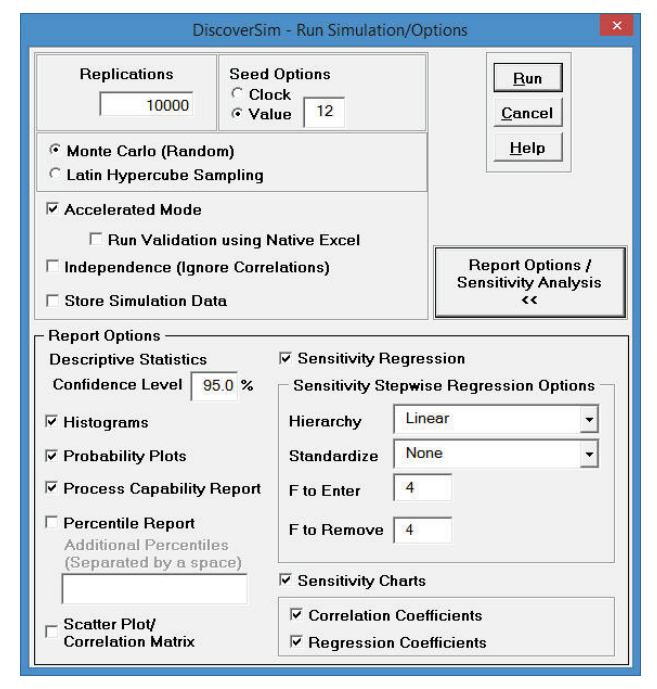Click Run

95. Click on the Sensitivity Correlations sheet:

96.97. Click on the Sensitivity Regression sheet to view the R-Square percent contribution to variation:

98.The sensitivity charts confirm that Project E is the dominant “X” factor. This is a high impact project, but is also a difficult project with a probability of success of only 0.5 and a large variation (min = 2000, max = 2500). If possible, this project might be split into smaller, lower risk (higher probability of success) projects with less variation.

99. Optional Insert DSIM Function: As an alternative to Maximize CpL as shown above, we could also Maximize Mean Savings subject to an added constraint that the Standard Deviation of Savings be < 500. Click on cell Q27 and select DiscoverSim > DSIM Function:

100.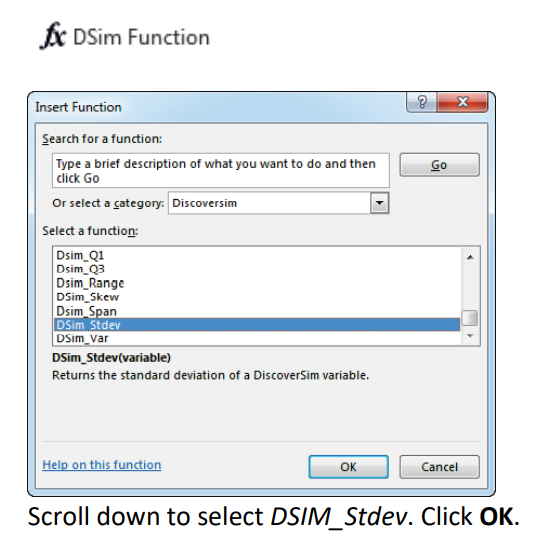101. Select the Output Q24 as the DSIM Variable.

102.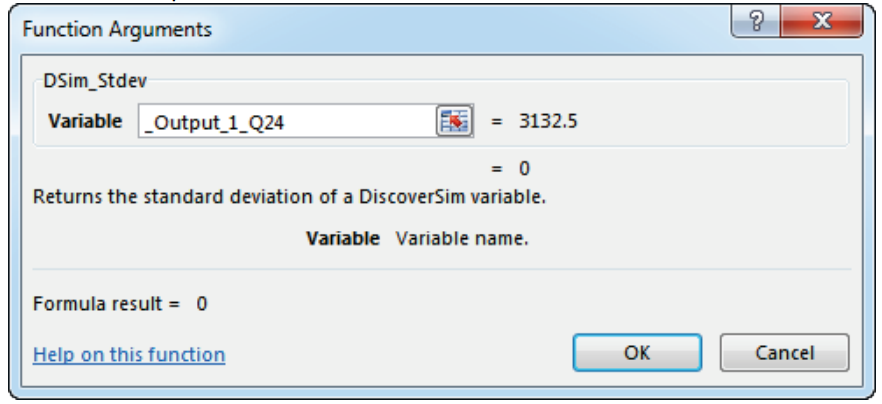The DiscoverSim Range Name for Q24 is _Output_1_Q24. Click OK.

103. The DSIM function formula is now in cell = DSim_Stdev(_Output_1_Q24)
104. Now we will add a constraint at cell O27 that references the DSIM function. Click on cell O27. Select Constraint:

105.106. Enter the Constraint Name as “StDev_Constraint”. Enter Q27 in the “Left Hand Side” (LHS) or click the LHS cell referenceand select Q27. Select <. Enter 500 in the “Right Hand Side” (RHS).

107.108. Click OK. Review the comment at cell O27:

109.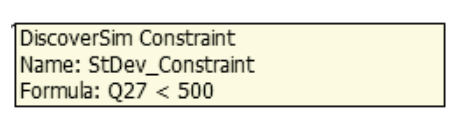The cell display is “TRUE” since the initial value for Q27 is zero which obviously satisfies the constraint.110. With all of the Input Control values set to 1, select DiscoverSim > Run Optimization:

111.112. Select “Maximize” for Optimization Goal, “Weighted Sum” for Multiple Output Metric and “Mean” for Statistic. Select Seed Value and enter “12,” in order to replicate the optimization results given below. Set Replications to 1000 to reduce the optimization time. All other settings will be the defaults as shown:

113.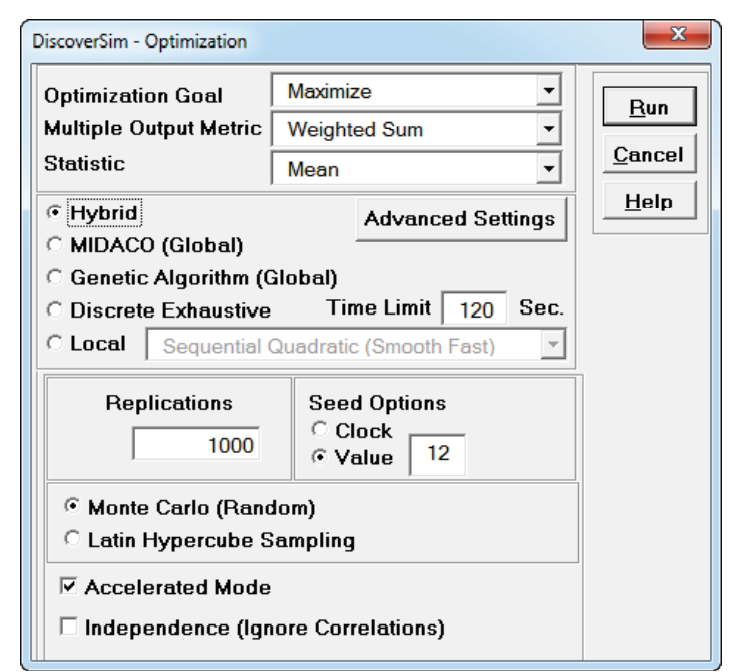Click Run.

114. The final optimal parameter values are given as:

115.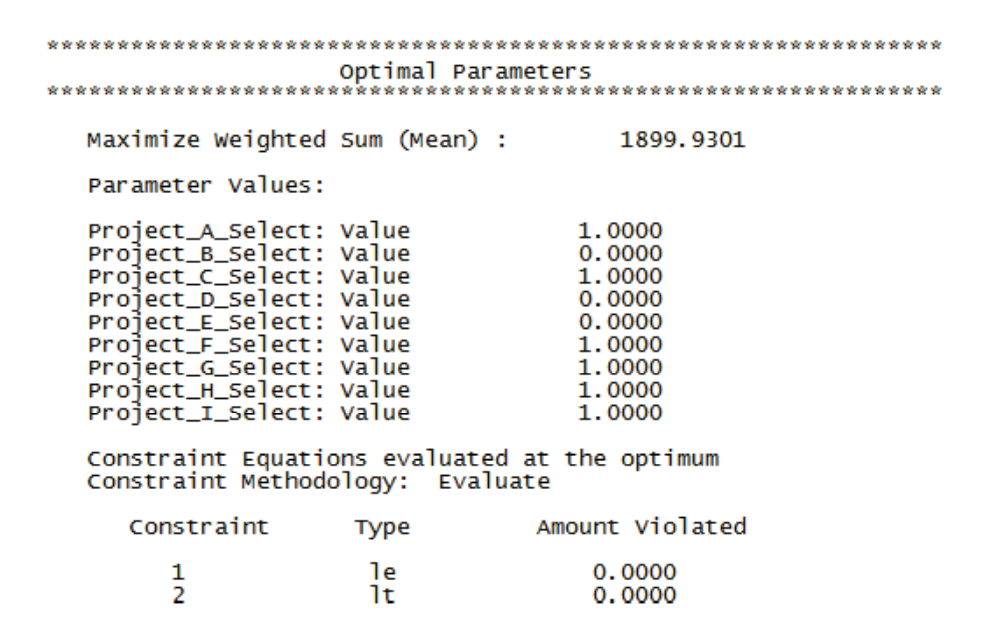Projects A, C, F, G, H, and I have been selected giving a predicted Mean of 1899.9. These are exactly the same projects that were selected above with maximize PpL.

116. You are prompted to paste the optimal values into the spreadsheet:
117.Since we have already analyzed this project selection, we will not pursue this further. Click No.

118. The use of Insert DSIM Function as shown provides flexibility in specifying the optimization objective function. For example in a multiple output scenario, you can Maximize the Mean of Output 1, with a constraint that Output 2 Ppk process capability must be > 1.

# Web Demos

Our CTO and Co-Founder, John Noguera, regularly hosts free Web Demos featuring SigmaXL and DiscoverSim